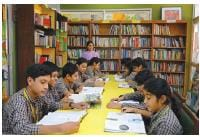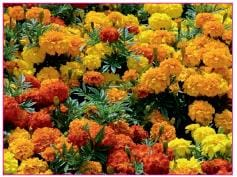# Case Based Questions Test: Real Numbers - 1

## 10 Questions MCQ Test NCERT Mathematics for CAT Preparation | Case Based Questions Test: Real Numbers - 1

Description
Attempt Case Based Questions Test: Real Numbers - 1 | 10 questions in 20 minutes | Mock test for Class 10 preparation | Free important questions MCQ to study NCERT Mathematics for CAT Preparation for Class 10 Exam | Download free PDF with solutions
QUESTION: 1

### Direction: Read the following text and answer the following questions on the basis of the same: To enhance the reading skills of grade X students, the school nominates you and two of your friends to set up a class library. There are two sections- section A and section B of grade X. There are 32 students in section A and 36 students in section B.If p and q are positive integers such that p = ab2 and q = a2b, where a, b are prime numbers, then the LCM (p, q) is

Solution:

Given, p = ab2 = a × b × b

q = a2b = a × a × b

LCM of (p, q) = a2b2

QUESTION: 2

### Direction: Read the following text and answer the following questions on the basis of the same: To enhance the reading skills of grade X students, the school nominates you and two of your friends to set up a class library. There are two sections- section A and section B of grade X. There are 32 students in section A and 36 students in section B.If the product of two positive integers is equal to the product of their HCF and LCM is true then, the HCF (32 , 36) is

Solution:

Prime factor 32 is 2 x 2 x 2 x 2 x 2

Prime factor 36 is 2 x 2 x 3 x 3

HCF is 2 x 2 i.e 4

HCF of 32 and 36 is 4.

QUESTION: 3

### Direction: Read the following text and answer the following questions on the basis of the same:To enhance the reading skills of grade X students, the school nominates you and two of your friends to set up a class library. There are two sections- section A and section B of grade X. There are 32 students in section A and 36 students in section B.7 × 11 × 13 × 15 + 15 is a

Solution: 7 × 11 × 13 × 15 + 15 is composite number.

Take 15 common

15 ( 7 × 11 × 13 + 1)

So, given number has factor other than 1 and itself so it is a composite number.

QUESTION: 4

Direction: Read the following text and answer the following questions on the basis of the same:

To enhance the reading skills of grade X students, the school nominates you and two of your friends to set up a class library. There are two sections- section A and section B of grade X. There are 32 students in section A and 36 students in section B.What is the minimum number of books you will acquire for the class library, so that they can be distributed equally among students of Section A or Section B?

Solution: We have to find the LCM of 32 and 36.

LCM(32, 36) = 25 × 9 = 288

Hence, the minimum number of books required to distribute equally among students of section A and section B are 288.

QUESTION: 5

Direction: Read the following text and answer the following questions on the basis of the same:

To enhance the reading skills of grade X students, the school nominates you and two of your friends to set up a class library. There are two sections- section A and section B of grade X. There are 32 students in section A and 36 students in section B.36 can be expressed as a product of its primes as

Solution: The product of its prime factors are:-

36 = 2 × 2 × 3 × 3

= 22 × 33

QUESTION: 6

Direction: Read the following text and answer the following questions on the basis of the same: A garden consists of 135 rose plants planted in certain number of columns. There are another set of 225 marigold plants, which is to be planted in the same number of columns.Find the sum of exponents of the prime factors of total number of plants.

Solution: Total number of plants = 135 + 225 = 360

The prime factors of 360 = 2 × 2 × 2 × 3 × 3 × 5

= 23 × 32 × 51

∴ Sum of exponents = 3 + 2 + 1 = 6.

QUESTION: 7

Direction: Read the following text and answer the following questions on the basis of the same: A garden consists of 135 rose plants planted in certain number of columns. There are another set of 225 marigold plants, which is to be planted in the same number of columns.Find the total number of plants

Solution: Total number of plants 135 + 225 = 360 plants
QUESTION: 8

Direction: Read the following text and answer the following questions on the basis of the same: A garden consists of 135 rose plants planted in certain number of columns. There are another set of 225 marigold plants, which is to be planted in the same number of columns.What is total numbers of row in which they can be planted

Solution: Number of rows of Rose plants = 135/45 = 3

Number of rows of marigold plants = 225/45 = 5

Total number of rows = 3 + 5 = 8

QUESTION: 9

Direction: Read the following text and answer the following questions on the basis of the same: A garden consists of 135 rose plants planted in certain number of columns. There are another set of 225 marigold plants, which is to be planted in the same number of columns.What is the maximum number of columns in which they can be planted ?

Solution: No. of rose plants = 135

No. of marigold plants = 225

The maximum number of columns in which they can be planted

= HCF of 135 and 225

∴ Prime factors of 135 = 3 × 3 × 3 × 5

and 225 = 3 × 3 × 5 × 5

∴ Prime factors of 135 = 3 × 3 × 5 = 45.

QUESTION: 10

Direction: Read the following text and answer the following questions on the basis of the same: A garden consists of 135 rose plants planted in certain number of columns. There are another set of 225 marigold plants, which is to be planted in the same number of columns.Find the sum of exponents of the prime factors of the maximum number of columns in which they can be planted.

Solution: We have proved that the maximum number of columns = 45

So, prime factors of 45

= 3 × 3 × 5 = 32 × 51

∴ Sum of exponents = 2 + 1 = 3.Use Code STAYHOME200 and get INR 200 additional OFF Use Coupon Code